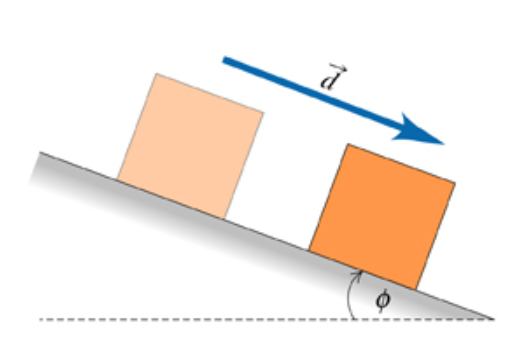# Problem: A box of weight w=2.0N accelerates down a rough plane that is inclined at an angle Φ =30° above the horizontal, as shown (Figure 6) . The normal force acting on the box has a magnitude n=1.7 N, the coefficient of kinetic friction between the box and the plane is μk=0.30, and the displacement d? of the box is 1.8 m down the inclined plane.Part a. What is the work Wn done on the box by the normal force?Express your answers in joules to two significant figures.Part b. What is the work Wfk done on the box by the force of kinetic friction?Express your answers in joules to two significant figures.

###### FREE Expert Solution

Work in done by force in an incline:

$\overline{){\mathbf{W}}{\mathbf{=}}{\mathbf{F}}{\mathbf{d}}{\mathbf{c}}{\mathbf{o}}{\mathbf{s}}{\mathbf{\theta }}}$

Work done by force of kinetic friction:

$\overline{){\mathbf{W}}{\mathbf{=}}{\mathbf{-}}{{\mathbf{\mu }}}_{{\mathbf{k}}}{\mathbf{n}}{\mathbf{d}}}$

Part a

The normal force is perpendicular to the displacement of the box.

Therefore, θ = 90°

93% (390 ratings)###### Problem Details

A box of weight w=2.0N accelerates down a rough plane that is inclined at an angle Φ =30° above the horizontal, as shown (Figure 6) . The normal force acting on the box has a magnitude n=1.7 N, the coefficient of kinetic friction between the box and the plane is μk=0.30, and the displacement d? of the box is 1.8 m down the inclined plane.

Part a. What is the work Wn done on the box by the normal force?

Part b. What is the work Wfk done on the box by the force of kinetic friction?